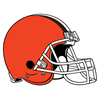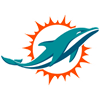DraftKings AFC Championship Odds
+200
2 to 1Bills
29.9% implied probability

+600
6 to 1Ravens
12.8% implied probability

+650
6.5 to 1Chiefs
12.0% implied probability

+800
8 to 1Chargers
10.0% implied probability

+800
8 to 1Titans
10.0% implied probability

+1200
12 to 1Browns
6.9% implied probability

+1600
16 to 1Bengals
5.3% implied probability

+2200
22 to 1Raiders
3.9% implied probability

+2800
28 to 1Colts
3.1% implied probability

+5000
50 to 1Broncos
1.8% implied probability

+5000
50 to 1Patriots
1.8% implied probability

+5000
50 to 1Steelers
1.8% implied probability

+25000
250 to 1Dolphins
0.4% implied probability

+50000
500 to 1Texans
0.2% implied probability

+50000
500 to 1Jaguars
0.2% implied probability

+50000
500 to 1Jets
0.2% implied probability